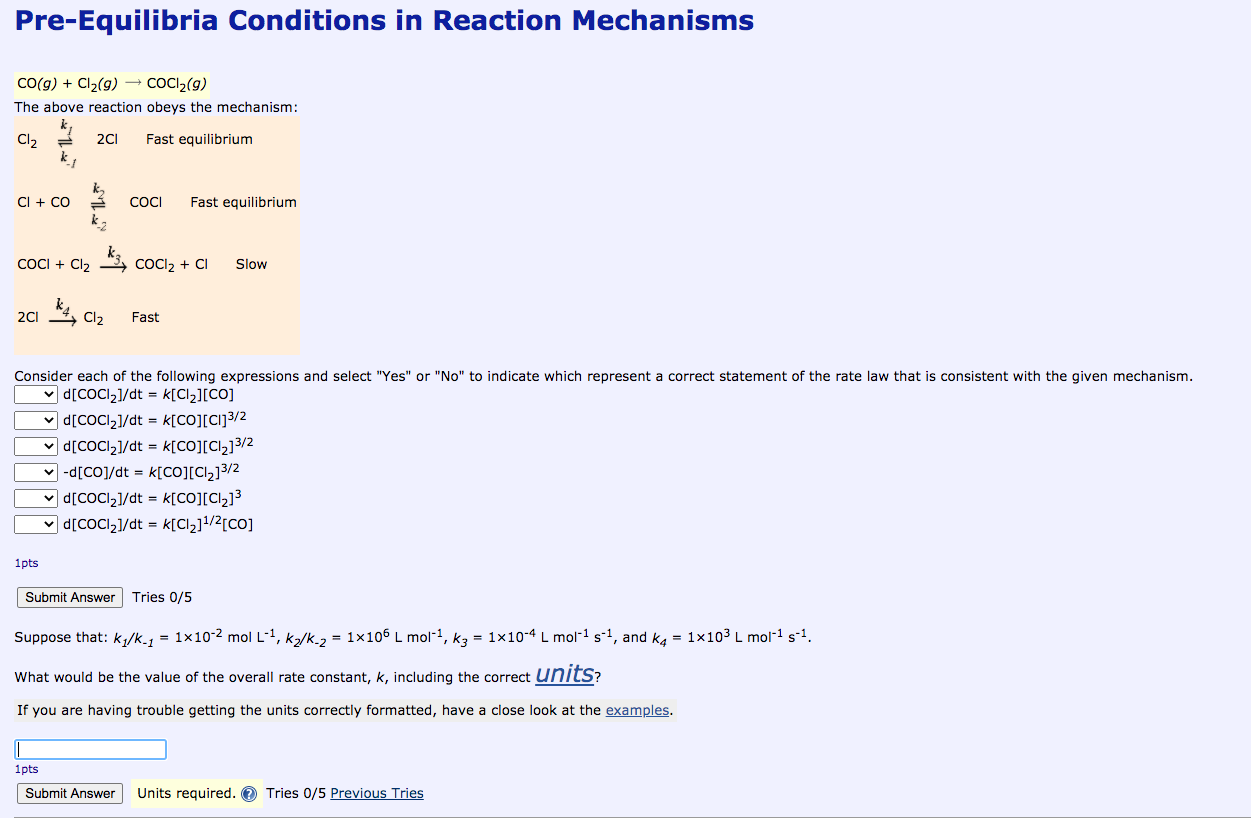# Question Solved1 AnswerPre-Equilibria Conditions in Reaction Mechanisms CO(g) + Cl2(g) → CoCl2(9) The above reaction obeys the mechanism: Cl2 2C1 Fast equilibrium k Cl + CO COCI Fast equilibrium COCI + Cl2 ki, COCIZ + C1 Slow 2C1 Cl2 Fast Consider each of the following expressions and select "Yes" or "No" to indicate which represent a correct statement of the rate law that is consistent with the given mechanism. d[CoCl2]/dt = k[Cl2][CO] v d[COCI2]/dt = k[CO][C1]3/2 v [CoCl21/dt = K[CO][Cl]3/2 -d[Co]/dt = k[CO](Cl2]3/2 v [CoCl21/dt = K[CO][Cl]3 v d[COCI2]/dt = k[Cl2]1/2[co] 1 pts Submit Answer Tries 0/5 Suppose that: kq/k_1 = 1x10-2 mol L-4, Kz/k.2 = 1x106 L mol-2, kg = 1x10-4 L mol-1 3-1, and ka = 1x103 L mol-1 5-1. What would be the value of the overall rate constant, k, including the correct units? If you are having trouble getting the units correctly formatted, have a close look at the examples. 1 pts Submit Answer Units required. Tries 0/5 Previous TriesTranscribed Image Text: Pre-Equilibria Conditions in Reaction Mechanisms CO(g) + Cl2(g) → CoCl2(9) The above reaction obeys the mechanism: Cl2 2C1 Fast equilibrium k Cl + CO COCI Fast equilibrium COCI + Cl2 ki, COCIZ + C1 Slow 2C1 Cl2 Fast Consider each of the following expressions and select "Yes" or "No" to indicate which represent a correct statement of the rate law that is consistent with the given mechanism. d[CoCl2]/dt = k[Cl2][CO] v d[COCI2]/dt = k[CO][C1]3/2 v [CoCl21/dt = K[CO][Cl]3/2 -d[Co]/dt = k[CO](Cl2]3/2 v [CoCl21/dt = K[CO][Cl]3 v d[COCI2]/dt = k[Cl2]1/2[co] 1 pts Submit Answer Tries 0/5 Suppose that: kq/k_1 = 1x10-2 mol L-4, Kz/k.2 = 1x106 L mol-2, kg = 1x10-4 L mol-1 3-1, and ka = 1x103 L mol-1 5-1. What would be the value of the overall rate constant, k, including the correct units? If you are having trouble getting the units correctly formatted, have a close look at the examples. 1 pts Submit Answer Units required. Tries 0/5 Previous Tries
More
Transcribed Image Text: Pre-Equilibria Conditions in Reaction Mechanisms CO(g) + Cl2(g) → CoCl2(9) The above reaction obeys the mechanism: Cl2 2C1 Fast equilibrium k Cl + CO COCI Fast equilibrium COCI + Cl2 ki, COCIZ + C1 Slow 2C1 Cl2 Fast Consider each of the following expressions and select "Yes" or "No" to indicate which represent a correct statement of the rate law that is consistent with the given mechanism. d[CoCl2]/dt = k[Cl2][CO] v d[COCI2]/dt = k[CO][C1]3/2 v [CoCl21/dt = K[CO][Cl]3/2 -d[Co]/dt = k[CO](Cl2]3/2 v [CoCl21/dt = K[CO][Cl]3 v d[COCI2]/dt = k[Cl2]1/2[co] 1 pts Submit Answer Tries 0/5 Suppose that: kq/k_1 = 1x10-2 mol L-4, Kz/k.2 = 1x106 L mol-2, kg = 1x10-4 L mol-1 3-1, and ka = 1x103 L mol-1 5-1. What would be the value of the overall rate constant, k, including the correct units? If you are having trouble getting the units correctly formatted, have a close look at the examples. 1 pts Submit Answer Units required. Tries 0/5 Previous Tries